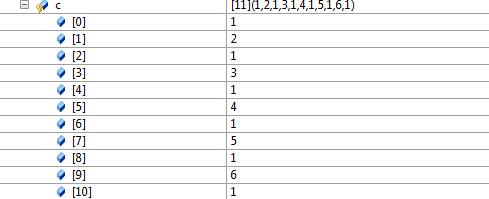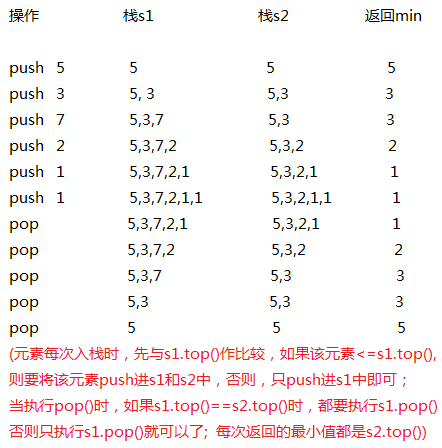实现一个栈，要求push,pop,Min的操作时间复杂度为O(1)

#include<assert.h>
#include<stack>
#include<iostream>
using namespace std;

template<typename T>
class Stack
{
public:
void Push(const T& d)
{
//栈中为空或要压入的元素小于等于栈顶元素，则将该元素压入两次
if(_s.empty() || d<=_s.top())
{
_s.push(d);
_s.push(d);
}
else
{
T mindata=_s.top();     //先将最小值保存下来
_s.push(d);
_s.push(mindata);      //根据栈的“后进先出”的道理
}
}
void Pop()
{
//对空栈pop操作非法
assert(!_s.empty());
_s.pop();               //在Push时保持了top()元素为最小值
_s.pop();

}
T& Min()
{
if(_s.empty())
{
cout<<"Search Min Error"<<endl;
exit(1);
}
return _s.top();
}
private:
stack<T> _s;
};

void test()
{
Stack<int> s;
s.Push(9);
s.Push(2);
s.Push(6);
s.Push(4);
cout<<"min-value:"<<s.Min()<<endl;
s.Pop();
}

int main()
{
test();
system("pause");
return 0;
}template<typename T>
class Stack
{
public:
void Push(const T& d);
void Pop();
T& Min();
T& Top();
private:
stack<T> s;  //存放所有数据
stack<T> min;  //存放较小数据
};
template<typename T>
void Stack<T>::Push(const T& d)
{
s.push(d);
if(min.empty() || d<=min.top())
{
min.push(d);
}
}
template<typename T>
void Stack<T>::Pop()
{
assert(!s.empty());
if(s.top()==min.top())
{
min.pop();
}
s.pop();
}
template<typename T>
T& Stack<T>::Min()
{
return min.top();
}
void test()
{
Stack<int> s;
s.Push(5);
s.Push(3);
s.Push(7);
s.Push(2);
s.Push(1);
s.Push(1);
s.Pop();
cout<<s.Min()<<endl;
s.Pop();
cout<<s.Min()<<endl;
s.Pop();
cout<<s.Min()<<endl;
s.Pop();
cout<<s.Min()<<endl;
s.Pop();
cout<<s.Min()<<endl;
s.Pop();
}

1.为什么push时的条件是d<=min.top(),而不是d<min.top()？

2.该题目的两种解决方案都是以空间换时间，那么如果没有时间复杂度的要求，该如何做到解决空间浪费的问题？